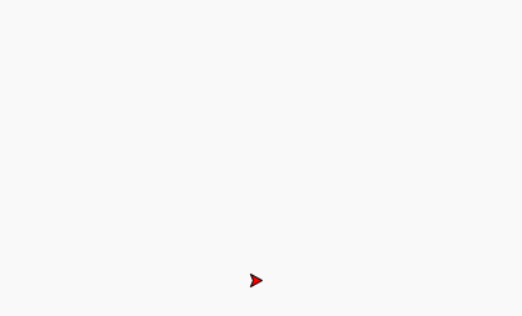Open in App
Not now

# Draw Heart Using Turtle Graphics in Python

• Difficulty Level : Medium
• Last Updated : 08 Jul, 2020

Turtle is an inbuilt module in Python. It provides:

1. Drawing using a screen (cardboard).
2. Turtle (pen).

To draw something on the screen, we need to move the turtle (pen) and to move the turtle, there are some functions like the forward(), backward(), etc

Prerequisite: Turtle Programming Basics

### Draw Heart Using Turtle Graphics

In this section, we will discuss how to draw Heart using Turtle Graphics.

Approach:

1. Import Turtle
2. Make Turtle Object
3. Define a method to draw a curve with simple forward and left moves
4. Define a method to draw the full heart and fill the red color in it
5. Define a method to display some text by setting position
6. Call all the methods in main section.

Code:

## python3

 `# Import turtle package``import` `turtle`` ` `# Creating a turtle object(pen)``pen ``=` `turtle.Turtle()`` ` `# Defining a method to draw curve``def` `curve():``    ``for` `i ``in` `range``(``200``):`` ` `        ``# Defining step by step curve motion``        ``pen.right(``1``)``        ``pen.forward(``1``)`` ` `# Defining method to draw a full heart``def` `heart():`` ` `    ``# Set the fill color to red``    ``pen.fillcolor(``'red'``)`` ` `    ``# Start filling the color``    ``pen.begin_fill()`` ` `    ``# Draw the left line``    ``pen.left(``140``)``    ``pen.forward(``113``)`` ` `    ``# Draw the left curve``    ``curve()``    ``pen.left(``120``)`` ` `    ``# Draw the right curve``    ``curve()`` ` `    ``# Draw the right line``    ``pen.forward(``112``)`` ` `    ``# Ending the filling of the color``    ``pen.end_fill()`` ` `# Defining method to write text``def` `txt():`` ` `    ``# Move turtle to air``    ``pen.up()`` ` `    ``# Move turtle to a given position``    ``pen.setpos(``-``68``, ``95``)`` ` `    ``# Move the turtle to the ground``    ``pen.down()`` ` `    ``# Set the text color to lightgreen``    ``pen.color(``'lightgreen'``)`` ` `    ``# Write the specified text in ``    ``# specified font style and size``    ``pen.write(``"GeeksForGeeks"``, font``=``(``      ``"Verdana"``, ``12``, ``"bold"``))`` ` ` ` `# Draw a heart``heart()`` ` `# Write text``txt()`` ` `# To hide turtle``pen.ht()`

Output:My Personal Notes arrow_drop_up